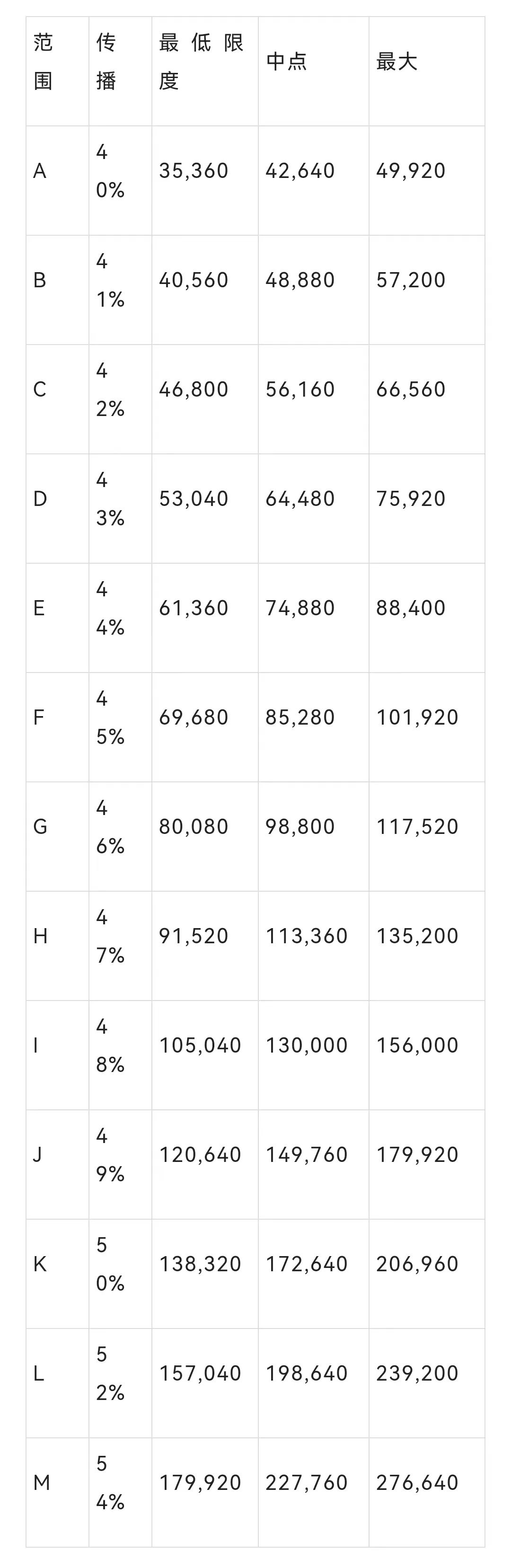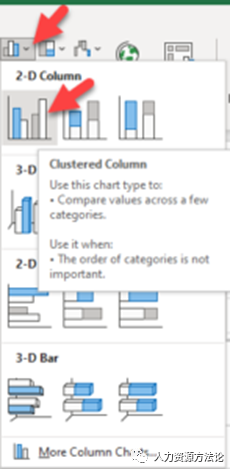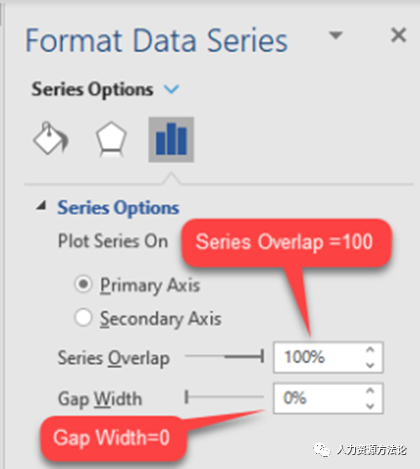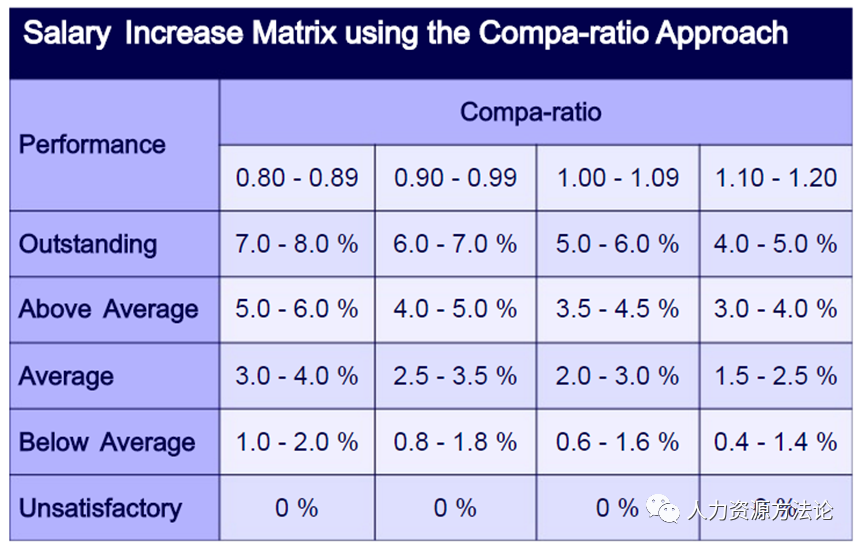HR必知的薪酬指标

### 1 1### 3 3

1、定义薪酬范围2、薪酬目标分位值

50 × (1 + 20%) = 60

3、薪酬范围中点值

46000 元 × (1 + 30%) = 59800 元

46000 元 × (1 – 10%) = 41400 元

4、薪酬级差5、范围小值

50000 元 / (1 + (40 % / 2) = 41667元（四舍五入）

6、范围[敏感词]值

[敏感词]值 = 小值 × (1 + 期望的范围扩展)

46667 × (1 + 40%) = 58333（四舍五入）7、薪资范围图8、工资差别

(74000 – 64,000) / 64000 = 15.63%

9、年度调整

9、强制分配

### 4 4

1、调薪比率（Compa-Ratio）

45000元 / 50000 元 = 0.92、薪资范围渗透

Compa-ratio 使用单个数据点，而范围渗透则考虑与整个薪酬范围相关的工资。

(45000 – 35000) / (65000 – 35000) = 33.33%

3、绿色圆圈

(38000 – 40000) / (60000 – 40000) = -0.1 (-10%)

4、红圈

(65000 – 40000) / (60000 – 40000) = 1.3 (130%)5、市场比率

60000 / (50000 × (1+15%)) = 1.04

6、地理城市差异

-END-

• 姓名
• *
• 联系方式
• 留言扫一扫/关注手机